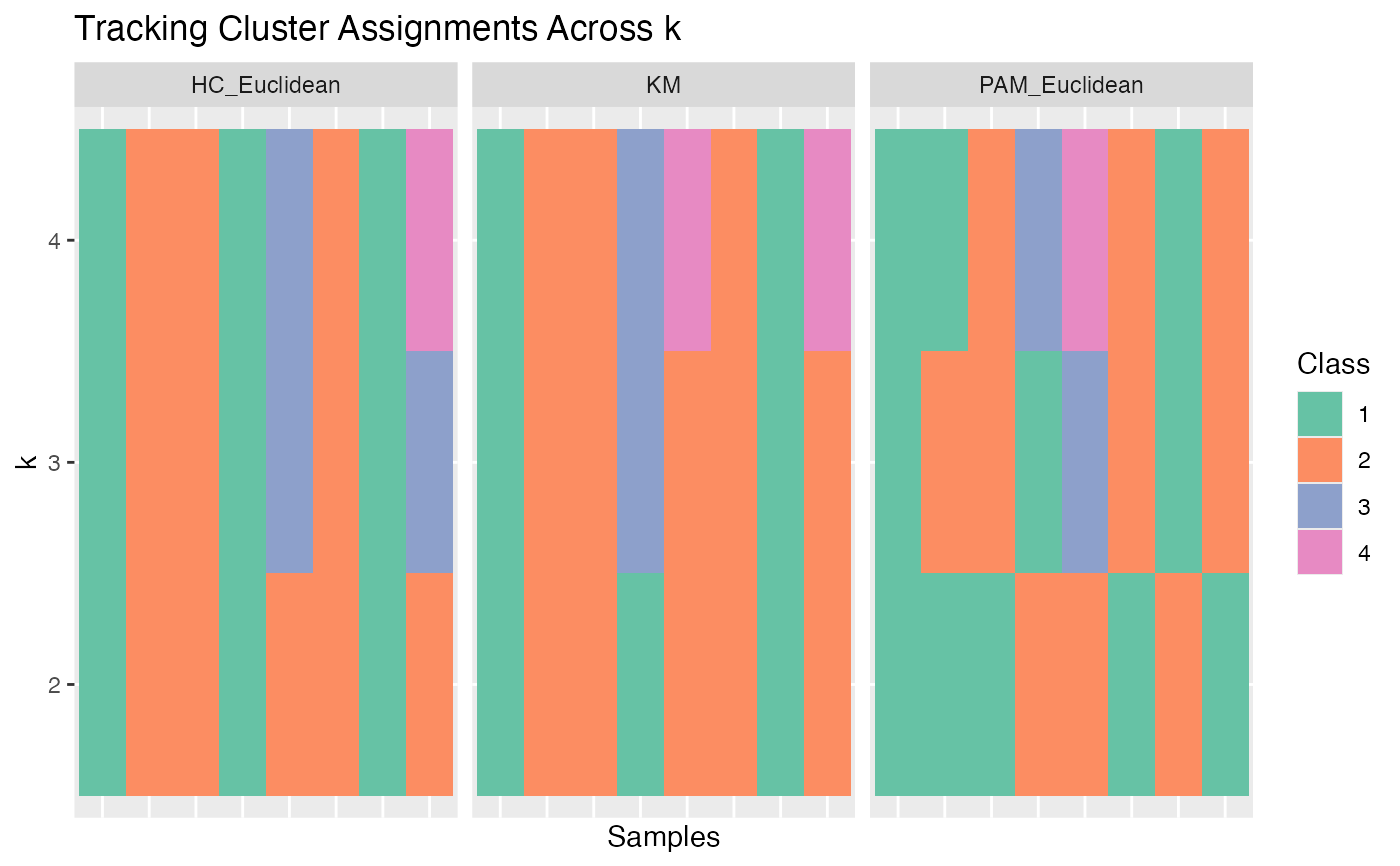Graph cumulative distribution function (CDF) graphs, relative change in area under CDF curves, heatmaps, and cluster assignment tracking plots.

graph_cdf(mat)

graph_delta_area(mat)

graph_heatmap(mat, main = NULL)

graph_tracking(cl)

graph_all(x)

## Arguments

mat

same as x, or a list of consensus matrices computed from x for faster results

main

heatmap title. If NULL (default), the titles will be taken from names in mat

cl

same as x, or a matrix of consensus classes computed from x for faster results

x

an object from consensus_cluster()

## Value

Various plots from graph_*{} functions. All plots are generated using ggplot, except for graph_heatmap, which uses NMF::aheatmap(). Colours used in graph_heatmap and graph_trackingutilize RColorBrewer::brewer.pal() palettes.

## Details

graph_cdf plots the CDF for consensus matrices from different algorithms. graph_delta_area calculates the relative change in area under CDF curve between algorithms. graph_heatmap generates consensus matrix heatmaps for each algorithm in x. graph_tracking tracks how cluster assignments change between algorithms. graph_all is a wrapper that runs all graphing functions.

Derek Chiu

## Examples

# Consensus clustering for 3 algorithms
library(ggplot2)
set.seed(911)
x <- matrix(rnorm(80), ncol = 10)
CC1 <- consensus_cluster(x, nk = 2:4, reps = 3,
algorithms = c("hc", "pam", "km"), progress = FALSE)

# Plot CDF
p <- graph_cdf(CC1)
#> Warning: separate_() was deprecated in tidyr 1.2.0.
#> Please use separate() instead.
#> This warning is displayed once every 8 hours.
#> Call lifecycle::last_lifecycle_warnings() to see where this warning was generated.
#> Warning: gather_() was deprecated in tidyr 1.2.0.
#> Please use gather() instead.
#> This warning is displayed once every 8 hours.
#> Call lifecycle::last_lifecycle_warnings() to see where this warning was generated.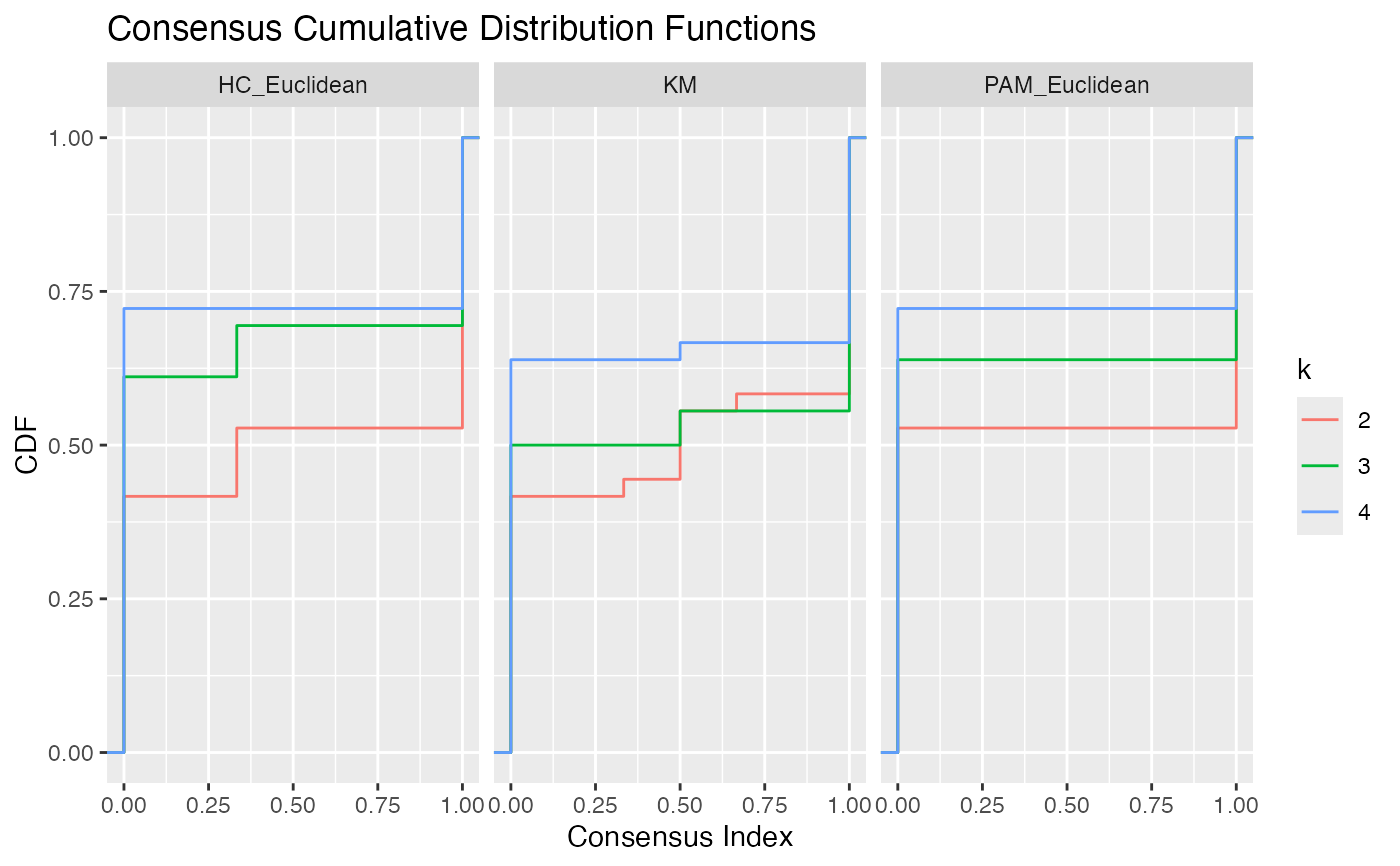# Change y label and add colours
p + labs(y = "Probability") + stat_ecdf(aes(colour = k)) +
scale_color_brewer(palette = "Set2")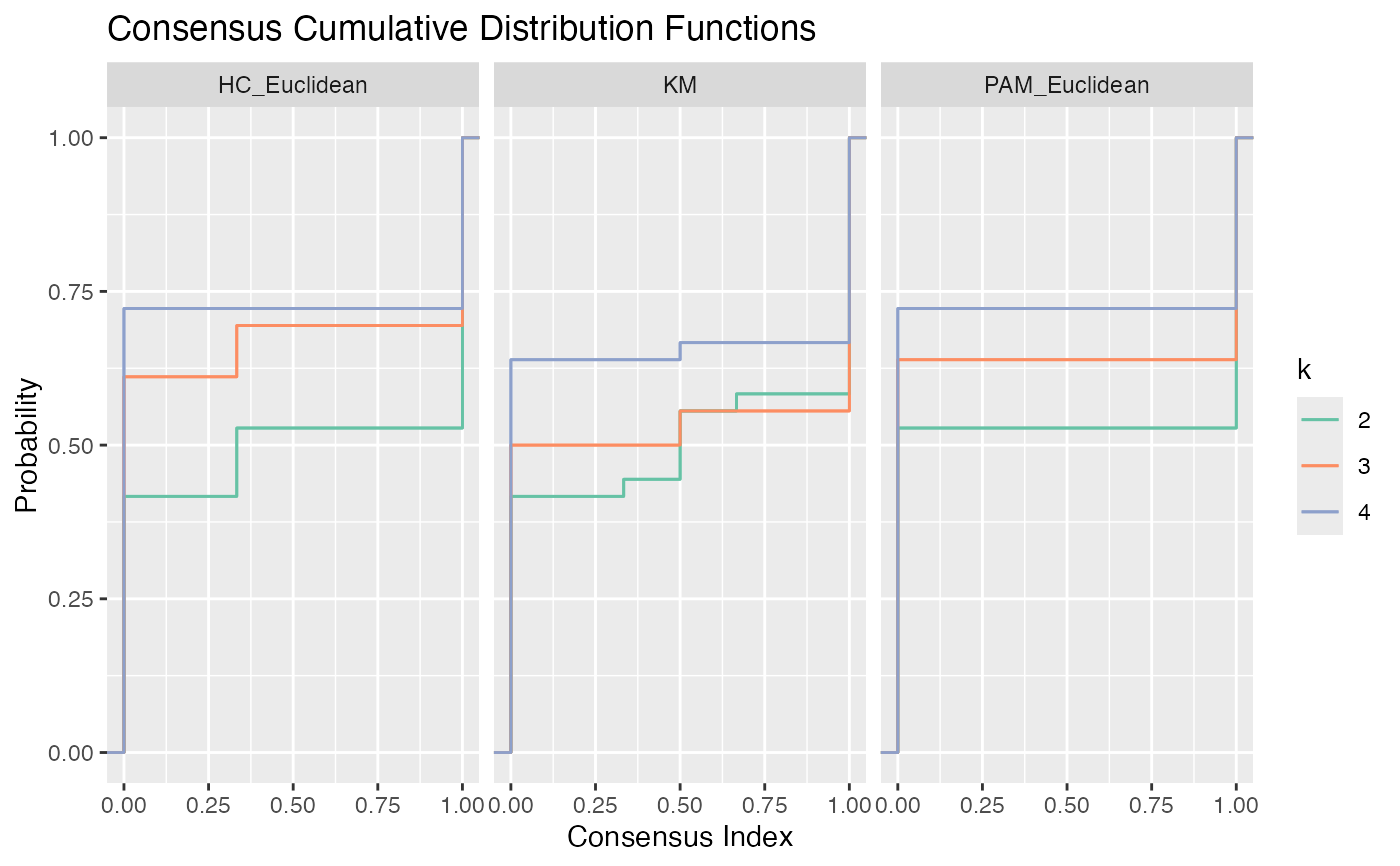# Delta Area
p <- graph_delta_area(CC1)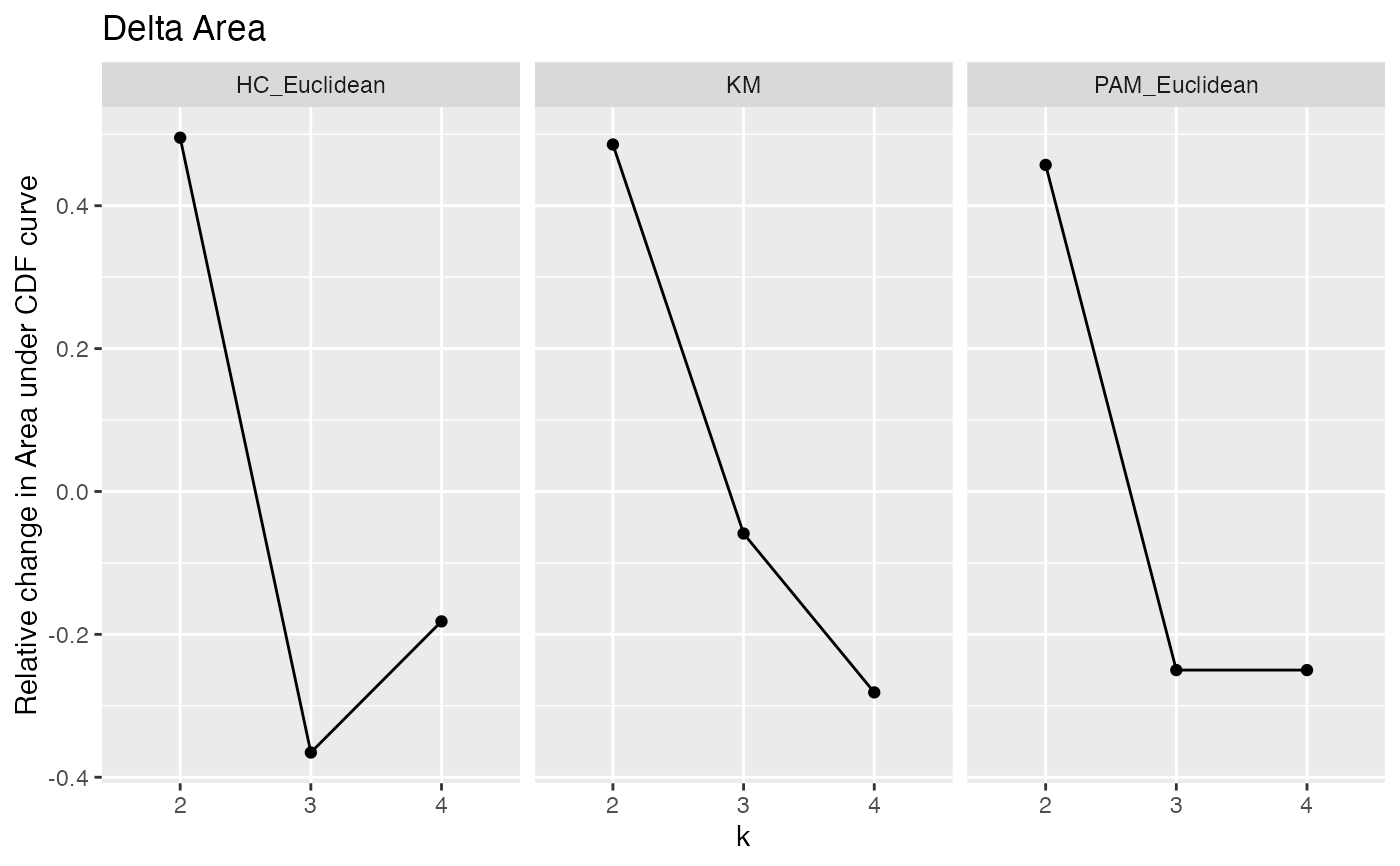# Heatmaps with column side colours corresponding to clusters
CC2 <- consensus_cluster(x, nk = 3, reps = 3, algorithms = "hc", progress =
FALSE)
graph_heatmap(CC2)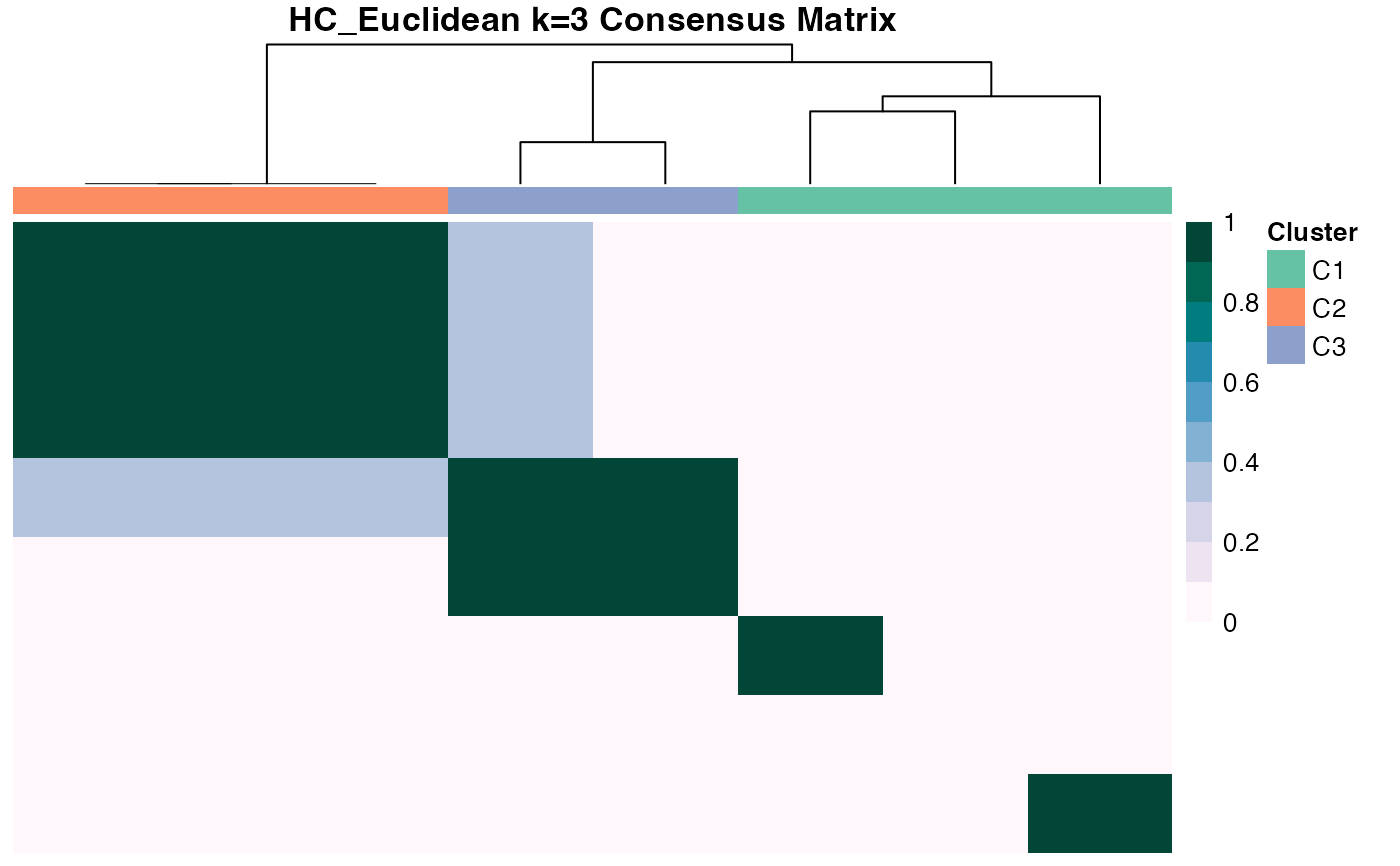# Track how cluster assignments change between algorithms
p <- graph_tracking(CC1)